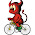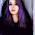Hi! Hope you're enjoying this blog. I have a new home at www.goldsborough.me. Be sure to also check by there for new posts <3

## Tuesday, July 16, 2013

### Tutorial: PyQt Calculator

What's the project?

Bonjour! Today I will show you how to make a PyQt Calculator. It won't be a scientific one, but you could certainly use this one instead of your operating system's one, so it'll be useful to you. When making small apps like these I find it especially statisfying if the app I made is actually useful to me, instead of making something just so you have it done, which you'll have to do of course to learn and progress in a language, but having projects like this one is just as good a way. Pragmatism all the way.

Building this calculator was a lot of fun, as it meant much more bare, raw logic-thinking than in my recent projects that I posted on here, where it's often just a lot of plug n play. You can either just copy the full code, or learn how to actually make it from scratch. This is what it will look like:

It does not include memory storage, but that would be fairly easy to do with a couple extra buttons and more global variables.

Let's get started

As always, here's the full code, ca. 300 lines:

```import sys
from PyQt4 import QtGui, QtCore
from PyQt4.QtCore import Qt
from math import sqrt

num = 0.0
newNum = 0.0
sumAll = 0.0
operator = ""

opVar = False
sumIt = 0

class Main(QtGui.QMainWindow):

def __init__(self):
QtGui.QMainWindow.__init__(self)
self.initUI()

def initUI(self):

self.line = QtGui.QLineEdit(self)
self.line.move(5,5)
self.line.setAlignment(Qt.AlignRight)
self.line.resize(200,25)

zero = QtGui.QPushButton("0",self)
zero.move(10,180)
zero.resize(35,30)

one = QtGui.QPushButton("1",self)
one.move(10,145)
one.resize(35,30)

two = QtGui.QPushButton("2",self)
two.move(50,145)
two.resize(35,30)

three = QtGui.QPushButton("3",self)
three.move(90,145)
three.resize(35,30)

four = QtGui.QPushButton("4",self)
four.move(10,110)
four.resize(35,30)

five = QtGui.QPushButton("5",self)
five.move(50,110)
five.resize(35,30)

six = QtGui.QPushButton("6",self)
six.move(90,110)
six.resize(35,30)

seven = QtGui.QPushButton("7",self)
seven.move(10,75)
seven.resize(35,30)

eight = QtGui.QPushButton("8",self)
eight.move(50,75)
eight.resize(35,30)

nine = QtGui.QPushButton("9",self)
nine.move(90,75)
nine.resize(35,30)

switch = QtGui.QPushButton("+/-",self)
switch.move(50,180)
switch.resize(35,30)
switch.clicked.connect(self.Switch)

point = QtGui.QPushButton(".",self)
point.move(90,180)
point.resize(35,30)
point.clicked.connect(self.pointClicked)

div = QtGui.QPushButton("/",self)
div.move(130,75)
div.resize(35,30)

mult = QtGui.QPushButton("*",self)
mult.move(130,110)
mult.resize(35,30)

minus = QtGui.QPushButton("-",self)
minus.move(130,145)
minus.resize(35,30)

plus = QtGui.QPushButton("+",self)
plus.move(130,180)
plus.resize(35,30)

sqrt = QtGui.QPushButton("√",self)
sqrt.move(170,75)
sqrt.resize(35,30)
sqrt.clicked.connect(self.Sqrt)

squared = QtGui.QPushButton("x²",self)
squared.move(170,110)
squared.resize(35,30)
squared.clicked.connect(self.Squared)

equal = QtGui.QPushButton("=",self)
equal.move(170,145)
equal.resize(35,65)
equal.clicked.connect(self.Equal)

c = QtGui.QPushButton("C",self)
c.move(145,35)
c.resize(60,30)
c.clicked.connect(self.C)

ce = QtGui.QPushButton("CE",self)
ce.move(77,35)
ce.resize(60,30)
ce.clicked.connect(self.CE)

back = QtGui.QPushButton("Back",self)
back.move(10,35)
back.resize(60,30)
back.clicked.connect(self.Back)

nums = [zero,one,two,three,four,five,six,seven,eight,nine]

ops = [back,c,ce,div,mult,minus,plus,equal]

rest = [switch,squared,sqrt,point]

for i in nums:
i.setStyleSheet("color:blue;")
i.clicked.connect(self.Nums)

for i in ops:
i.setStyleSheet("color:red;")

for i in ops[3:7]:
i.clicked.connect(self.Operator)

#---------Window settings --------------------------------

self.setGeometry(300,300,210,220)
self.setFixedSize(210,220)
self.setWindowTitle("")
self.setWindowIcon(QtGui.QIcon(""))
self.show()

def Nums(self):
global num
global newNum
global opVar

sender = self.sender()

newNum = int(sender.text())
setNum = str(newNum)

if opVar == False:
self.line.setText(self.line.text() + setNum)

else:
self.line.setText(setNum)
opVar = False

def pointClicked(self):
global opVar

if "." not in self.line.text():
self.line.setText(self.line.text() + ".")

def Switch(self):
global num

try:
num = int(self.line.text())

except:
num = float(self.line.text())

num = num - num * 2

numStr = str(num)

self.line.setText(numStr)

def Operator(self):
global num
global opVar
global operator
global sumIt

sumIt += 1

if sumIt > 1:
self.Equal()

num = self.line.text()

sender = self.sender()

operator = sender.text()

opVar = True

def Equal(self):
global num
global newNum
global sumAll
global operator
global opVar
global sumIt

sumIt = 0

newNum = self.line.text()

print(num)
print(newNum)
print(operator)

if operator == "+":
sumAll = float(num) + float(newNum)

elif operator == "-":
sumAll = float(num) - float(newNum)

elif operator == "/":
sumAll = float(num) / float(newNum)

elif operator == "*":
sumAll = float(num) * float(newNum)

print(sumAll)
self.line.setText(str(sumAll))
opVar = True

def Back(self):
self.line.backspace()

def C(self):
global newNum
global sumAll
global operator
global num

self.line.clear()

num = 0.0
newNum = 0.0
sumAll = 0.0
operator = ""

def CE(self):
self.line.clear()

def Sqrt(self):
global num

num = float(self.line.text())
n = sqrt(num)
num = n

self.line.setText(str(num))

def Squared(self):
global num

num = float(self.line.text())

n = num ** 2

num = n

self.line.setText(str(n))

def main():
app = QtGui.QApplication(sys.argv)
main= Main()
main.show()

sys.exit(app.exec_())

if __name__ == "__main__":
main()

```

It's rather large, so I'll only go over the most important parts.To get a plain window, copy the text I provide at the sidebar of my blog. Then, to start, import sqrt from math and Qt from PyQt.QtCore, also, create all those variables as we will need them later on.

You don't need to create all the buttons, just copy them. I chose to position them absolutely due to the simplicity of it.

```        nums = [zero,one,two,three,four,five,six,seven,eight,nine]

ops = [back,c,ce,div,mult,minus,plus,equal]

for i in nums:
i.setStyleSheet("color:blue;")
i.clicked.connect(self.Nums)

for i in ops:
i.setStyleSheet("color:red;")

for i in ops[3:7]:
i.clicked.connect(self.Operator)
```

Create two lists, nums and ops, and include all the number buttons in nums, and all the operators in ops. This is to connect all the numbers to the Nums function and also to style them, as it does make a difference whether all the buttons are the same color or not, even though it's just visual. Style them using setStyleSheet(), where you can include any other CSS that you want, but the color is the only important change. In the last for loop, I sliced the list so that only div, mult, minus and plus are concerned, and connected them to the function Operator, which we will make later.

```def Nums(self):
global opVar

sender = self.sender()

newNum = sender.text()

if opVar == False:
self.line.setText(self.line.text() + newNum)

else:
self.line.setText(newNum)
opVar = False
```

The first function. Since we will be using all those global variables, we need to call them first. Next, we need the function to detect which number is pressed, this is done with sender = self.sender(). We just assign the text of this button, which is the number, to the newNum variable. This function, as important as it might seem, is actually really only to display the number in the LineEdit, and does not influence the equation majorly. This opVar variable you see here, is the variable that makes sure that every time a number is pressed, it doesn't just display this number solely, but adds to the number you pressed before. Unless of course, an operator has been pressed and you want to type a new number.

```def pointClicked(self):

if "." not in self.line.text():
self.line.setText(self.line.text() + ".")
```

This function here, is the one that adds a comma, or point, to the number. It checks whether or not a comma is already in self.line.text(), and adds one if there is none.

```def Switch(self):
global num

try:
num = int(self.line.text())

except:
num = float(self.line.text())

num = num - num * 2

numStr = str(num)

self.line.setText(numStr)
```

This one changes the algebraic sign. The try and except clause is not really important, it just checks if the number is an int or a float. I did this because I didn't want a whole number to turn into a float when the +- sign is clicked, as it just wouldn't look good. Then, some simple math: may num = 5, then 5 - 5*2 = -5. The other way around, -5 - (-5*2) = 5. Then convert the number to a string again and set it to the LineEdit's text.

```    def Operator(self):
global num
global opVar
global operator
global sumIt

sumIt += 1

if sumIt > 1:
self.Equal()

num = self.line.text()

sender = self.sender()

operator = sender.text()

opVar = True
```

Now the function that all the operators are connected to. I'll explain the sumIt in a bit. Now, we get the number that is in the LineEdit, as this is the last time this number will be displayed, and assign it to num. Then, we need to detect where the signal is coming from and assign it's text, the operator's sign, to the global variable operator, which we will need later. Don't forget to set opVar to True now, as we want to type in a new number, and don't add numbers to the one in the LineEdit, after.

Now the sumIt part: Say we want to calculate 6*6*6 very quickly. Now, without this little bit of code, we would have to click equal after 6*6, and then calculate 36*6 again. Instead, we check whether an operator has been clicked more than one time, and if so, redirect it to the Equal function, so it automatically shows the current sum in the LineEdit. It will be set to 0 again once we actually click the equal button.

```    def Equal(self):
global num
global newNum
global sumAll
global operator
global opVar
global sumIt

sumIt = 0

newNum = self.line.text()

if operator == "+":
sumAll = float(num) + float(newNum)

elif operator == "-":
sumAll = float(num) - float(newNum)

elif operator == "/":
sumAll = float(num) / float(newNum)

elif operator == "*":
sumAll = float(num) * float(newNum)

self.line.setText(str(sumAll))
opVar = True
```

For the Equal function, call .. basically all the global variables. As said before, sumIt is set to 0 again. As this is the last time the second number of the equation is displayed, assign self.line.text() to newNum now. Next, we check what the operator's sign is, which we assigned to the variable operator in the Operator function, and finally do the actual equation with all the variables. Lastly, display the result in the text edit. with self.line.setText(str(sumAll)) and set opVar to True again.

```    def Back(self):
self.line.backspace()

def C(self):
global newNum
global sumAll
global operator
global num

self.line.clear()

num = 0.0
newNum = 0.0
sumAll = 0.0
operator = ""

def CE(self):
self.line.clear()
```

These functions here take care of the Back, C and CE buttons. I think what they do is rather clear. Back uses QLineEdits backspace function, C clears the LineEdit and sets all the variables to 0 and CE just clears the current display but doesn't delete the previous numbers.

```    def Sqrt(self):
global num

num = float(self.line.text())
n = sqrt(num)
num = n

self.line.setText(str(num))

def Squared(self):
global num

num = float(self.line.text())

n = num ** 2

num = n

self.line.setText(str(n))
```

These two functions do some simple math, Sqrt uses math.sqrt to get the squareroot of the number and Squared squares the number. Both numbers are then displayed in the LineEdit.

That should be it for this tutorial, please tell me of any bugs, or leave any kind of feedback in form of a comment beneath this post. Cheers!

#### 4 comments :

1.Hello,

First of all, what a great program!

I just didn't quite get the sender() function. Could you explain it in more details please.

Thank you!

2.Nice one..
I think you could add the widgets using a for loop

3.Free calculators for your every need. Find the right online calculator.

4.